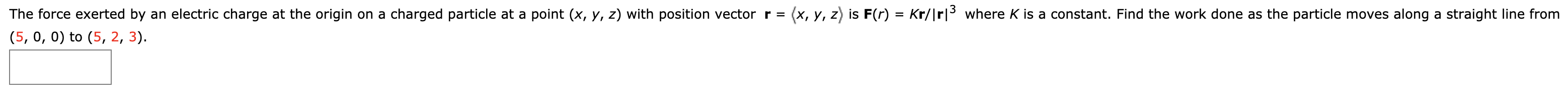# (x, y, z is F(r) = Kr/|rl3where K is a constant. Find the work done as the particle moves along a straight line from The force exerted by an electric charge at the origin on a charged particle at a point (x, y, z) with position vector r = (5, 0, 0) to (5, 2, 3)

Question

The force exerted by an electric charge at the origin on a charged particle at a point (xyz) with position vector

r =  x, y, z  is F(r) = Kr/|r|3

where K is a constant. Find the work done as the particle moves along a straight line from (5, 0, 0) to (5, 2, 3).help_outlineImage Transcriptionclose(x, y, z is F(r) = Kr/|rl3where K is a constant. Find the work done as the particle moves along a straight line from The force exerted by an electric charge at the origin on a charged particle at a point (x, y, z) with position vector r = (5, 0, 0) to (5, 2, 3) fullscreen